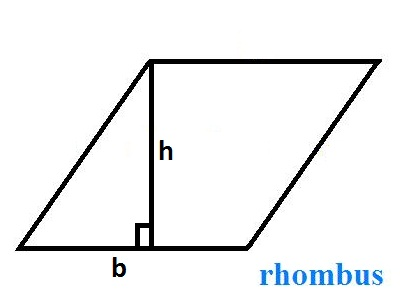# Rhombus Area Calculator and Perimeter Calculator

Rhombus area calculator tool is used to calculate area and perimeter of a rhombus, enter the length of the base (b) and height of the altitude (h) for calculations.

Area=base (b) x height (h)

Perimeter=4 x base (b)

## What is a Rhombus ?

A quadrilateral, all sides are equal in length, and opposite sides are parallel. The attributes indicate that a rhombus can also be classified as a parallelogram and all parallelograms are also classified as a trapezoid. The diagonals of a rhombus bisect one another but because they bisect each other at 90° angles, we call these diagonals perpendicular bisectors.

Area = b x h

Perimeter = 4b

#### Properties of Rhombus

• Opposite sides are congruent (they equal each other).
• Opposite angles are congruent (they equal each other).
• Consecutive angles are supplementary (they add up to 180).
• Diagonals bisect each other (the parts are equal).
• Diagonals perpendicular (the form right angles in the middle).
• Diagonals bisect angles (the angles are equal to each other).
• All four sides are congruent.
• The diagonals are NOT congruent.

## Latest Blog topics### Spreading Knowledge Across the World

USA - United States of America  Canada  United Kingdom  Australia  New Zealand  South America  Brazil  Portugal  Netherland  South Africa  Ethiopia  Zambia  Singapore  Malaysia  India  China  UAE - Saudi Arabia  Qatar  Oman  Kuwait  Bahrain  Dubai  Israil  England  Scotland  Norway  Ireland  Denmark  France  Spain  Poland  and  many more....